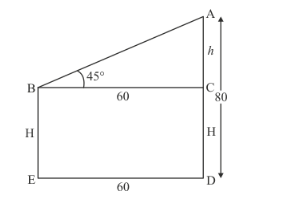# The horizontal distance between two trees of different heights is 60 m.

Question:

The horizontal distance between two trees of different heights is 60 m. The angle of depression of the top of the first tree when seen from the top of the second tree is 45°. If the height of the second tree is 80 m, find the height of the first tree.

Solution:

Let the difference between two trees be DE = 60 m and angle of depression of the first tree from the top to the top of the second tree is.

Let BE = H m, AC = h m, AD = 80m.

We have to find the height of the first tree

The corresponding figure is as follows$\ln \triangle A B C$

$\Rightarrow \quad \tan B=\frac{A C}{B C}$

$\Rightarrow \quad \tan 45^{\circ}=\frac{h}{60}$

$\Rightarrow \quad 1=\frac{h}{60}$

$\Rightarrow \quad h=60$

Since $H=80-h$

$\Rightarrow \quad H=80-60$

$\Rightarrow \quad H=20$

Hence the height of first tree is $20 \mathrm{~m}$.